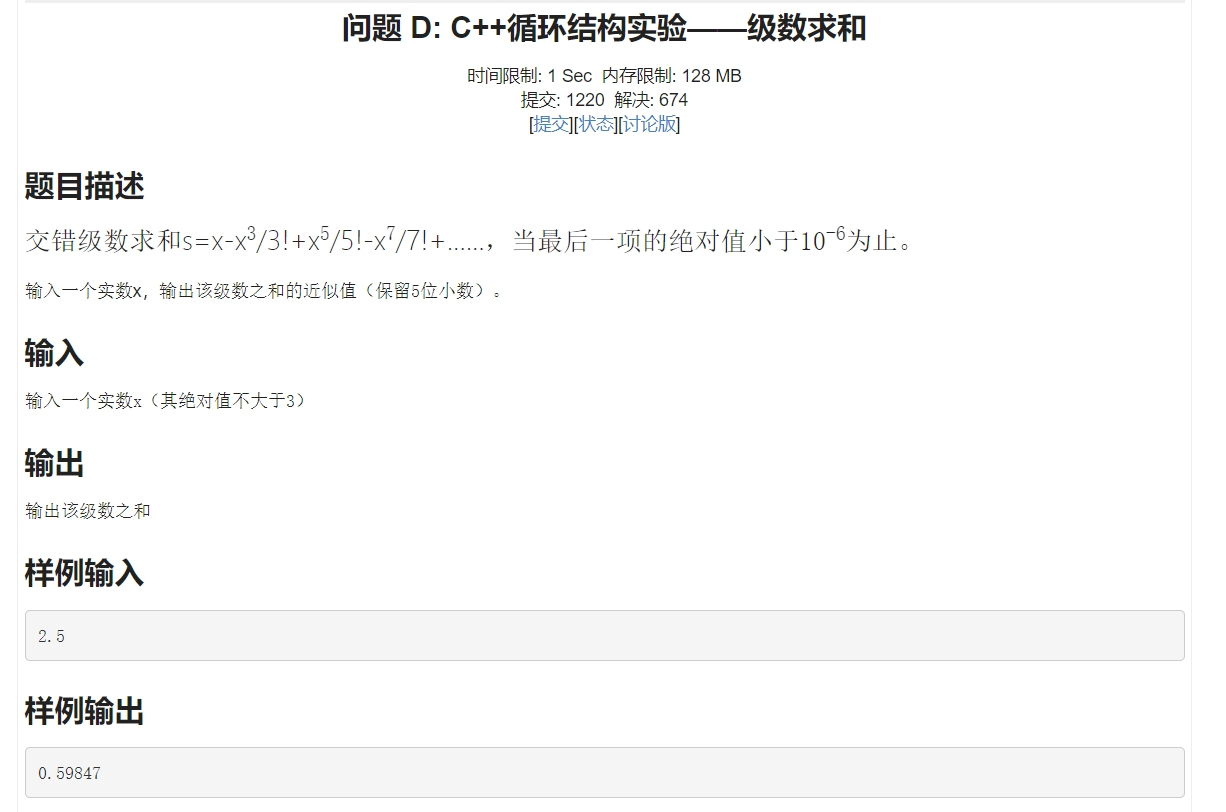2021-04-04 19:42

# 求c++大佬指点！！！• 点赞
• 写回答
• 关注问题
• 收藏
• 复制链接分享
• 邀请回答

#### 1条回答

• ``````#include<stdio.h>
#include<math.h>
int main(){
double x, y = 1;
scanf("%lf", &x);
double sum = 0, temp;
int flag = -1;
for(int i = 1; ; i++){
flag *= -1;
temp = pow(x,2*i-1);
if(i > 1)
y = y*(2*i-2)*(2*i-1);
if(temp/y < 0.000001)
break;
sum += flag*temp/y;
}
printf("%0.5lf", sum);
}``````
点赞 评论 复制链接分享